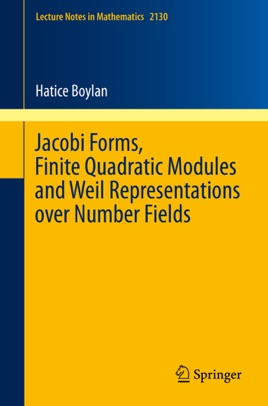• \$40.99

## Publisher Description

The new theory of Jacobi forms over totally real number fields introduced in this monograph is expected to give further insight into the arithmetic theory of Hilbert modular forms, its L-series, and into elliptic curves over number fields. This work is inspired by the classical theory of Jacobi forms over the rational numbers, which is an indispensable tool in the arithmetic theory of elliptic modular forms, elliptic curves, and in many other disciplines in mathematics and physics. Jacobi forms can be viewed as vector valued modular forms which take values in so-called Weil representations. Accordingly, the first two chapters develop the theory of finite quadratic modules and associated Weil representations over number fields. This part might also be interesting for those who are merely interested in the representation theory of Hilbert modular groups. One of the main applications is the complete classification of Jacobi forms of singular weight over an arbitrary totally real number field.